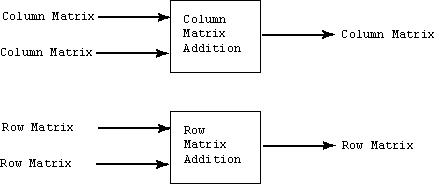`( 1, 2, 3 )T T`   =   `( 1, 2, 3 )`

Transposing twice gives you what you started with. This seems obvious right now, but later on when you are doing algebraic manipulation it might help to remember this.

# Column Matrix AdditionA column matrix added to another column matrix of the same dimension results in a column matrix (of the same dimension). A similar statement is true for row matrices.

Addition is done by adding corresponding elements of the input matrices to produce each corresponding element of the output matrix.

```( 42, -12 )T + ( 8, 24 )T = ( 50, 12 )T

( 32.98, -24.71, 9.392 )T + ( -32.98, +24.71, -9.392 )T = (0, 0, 0)T

( 9.2, -8.6, 3.21, 48.7 ) + ( -2.1, 4.3, 1.0, 2.3 ) = ( 7.1, -4.3, 4.21, 51.0 )

( 1, 2, 3 ) + ( 10, 20, 30 ) = ( 11, 22, 33 )
```

If a and b are matrices of the same type, then a + b = c means that each element ci = ai + bi

### QUESTION 3:

Do the following problem:

```( 2, -2 )T + ( 8,  6 )T =
```